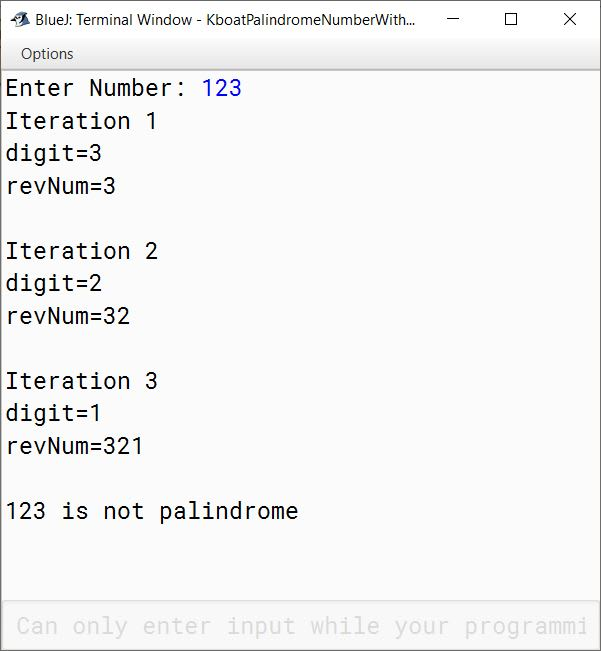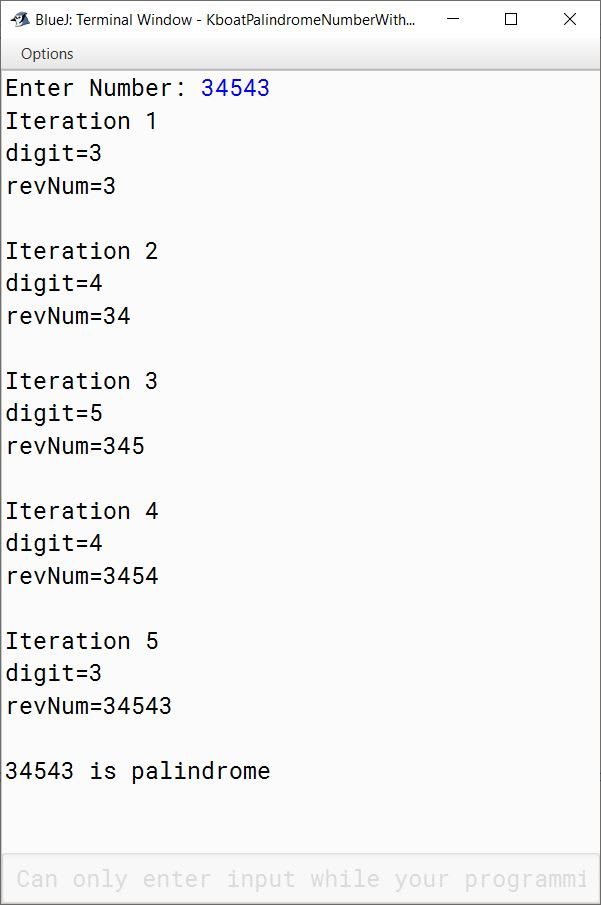PrevNextBack

# Palindrome Number Java Program

## What is a Palindrome Number?

In this lesson, we will look at palindrome number program. A number is said to be a palindrome if it remains the same when its digits are reversed. 747, 121, 48984, 34543 are some examples of palindrome numbers.

## Palindrome Number Program

This is the program to check if a given number is a palindrome or not.

```import java.util.Scanner;

{
public void checkPalindrome() {
Scanner in = new Scanner(System.in);
System.out.print("Enter Number: ");
int orgNum = in.nextInt();
int copyNum = orgNum;

int revNum = 0;

while(copyNum != 0) {
int digit = copyNum % 10;
copyNum /= 10;
revNum = revNum * 10 + digit;
}

if (revNum == orgNum)
System.out.println(orgNum + " is palindrome");
else
System.out.println(orgNum + " is not palindrome");

}
}```

Let's go over the program to understand it. In the below lines of the program:

```Scanner in = new Scanner(System.in);
System.out.print("Enter Number: ");
int orgNum = in.nextInt();
int copyNum = orgNum;```

we accept the number from the user with the help of scanner class and make a copy of it in variable `copyNum` to preserve the original input. After that we have the while loop:

```while(copyNum != 0) {
int digit = copyNum % 10;
copyNum /= 10;
revNum = revNum * 10 + digit;
}```

Inside the while loop, we are extracting each digit of the number into the int variable `digit`. The extraction of digits of a number is explained in detail here.

The line `revNum = revNum * 10 + digit;` is reversing the number digit-by-digit. To understand how this line is reversing the number, let us add a few statements to print the value of digit and revNum at each iteration of the while loop. Below is the program with the additional print statements added and its output:

```import java.util.Scanner;

{
public void checkPalindrome() {
Scanner in = new Scanner(System.in);
System.out.print("Enter Number: ");
int orgNum = in.nextInt();
int copyNum = orgNum;

int revNum = 0;
int itr = 1;

while(copyNum != 0) {
int digit = copyNum % 10;
copyNum /= 10;
revNum = revNum * 10 + digit;

/*
* for explanation
*/
System.out.println("Iteration " + itr++);
System.out.println("digit=" + digit);
System.out.println("revNum=" + revNum);
System.out.println();
}

if (revNum == orgNum)
System.out.println(orgNum + " is palindrome");
else
System.out.println(orgNum + " is not palindrome");

}
}```

#### OutputAs you can see in the output above, in the first iteration, the digit in the unit’s place which is 3 is extracted. Current value of revNum is zero, so the line `revNum = revNum * 10 + digit;` multiplies 0 with 10 and adds the value of variable `digit` to it effectively storing `digit` in `revNum`. `revNum` now becomes 3 which is shown in the output of the first iteration:

revNum = revNum * 10 + digit;
revNum = 0 * 10 + 3    [∵ digit = 3]
revNum = 0 + 3
revNum = 3

In the second iteration, the digit 2 is extracted. `revNum` is 3, multiplying it with 10 and adding 2 to it gives us 32 as shown in the output of the second iteration:

revNum = revNum * 10 + digit;
revNum = 3 * 10 + 2    [∵ digit = 2]
revNum = 30 + 2
revNum = 32

Notice that by the end of second iteration, we have reversed 2 digits of the number.

In the third iteration, the digit 1 is extracted. Current value of `revNum` is 32 and it is changed to 321 as shown in the output:

revNum = revNum * 10 + digit;
revNum = 32 * 10 + 1    [∵ digit = 1]
revNum = 320 + 1
revNum = 321

At this point, we have successfully reversed the number.

After third iteration, number becomes zero, so the while loop completes. Program control moves to the if-else after the loop. We compare the reversed number with the original number, if both are equal then number is a palindrome otherwise it is not.

Let us run the program with a palindrome number 34543 as input and verify that the program correctly identifies it as a palindromic number.